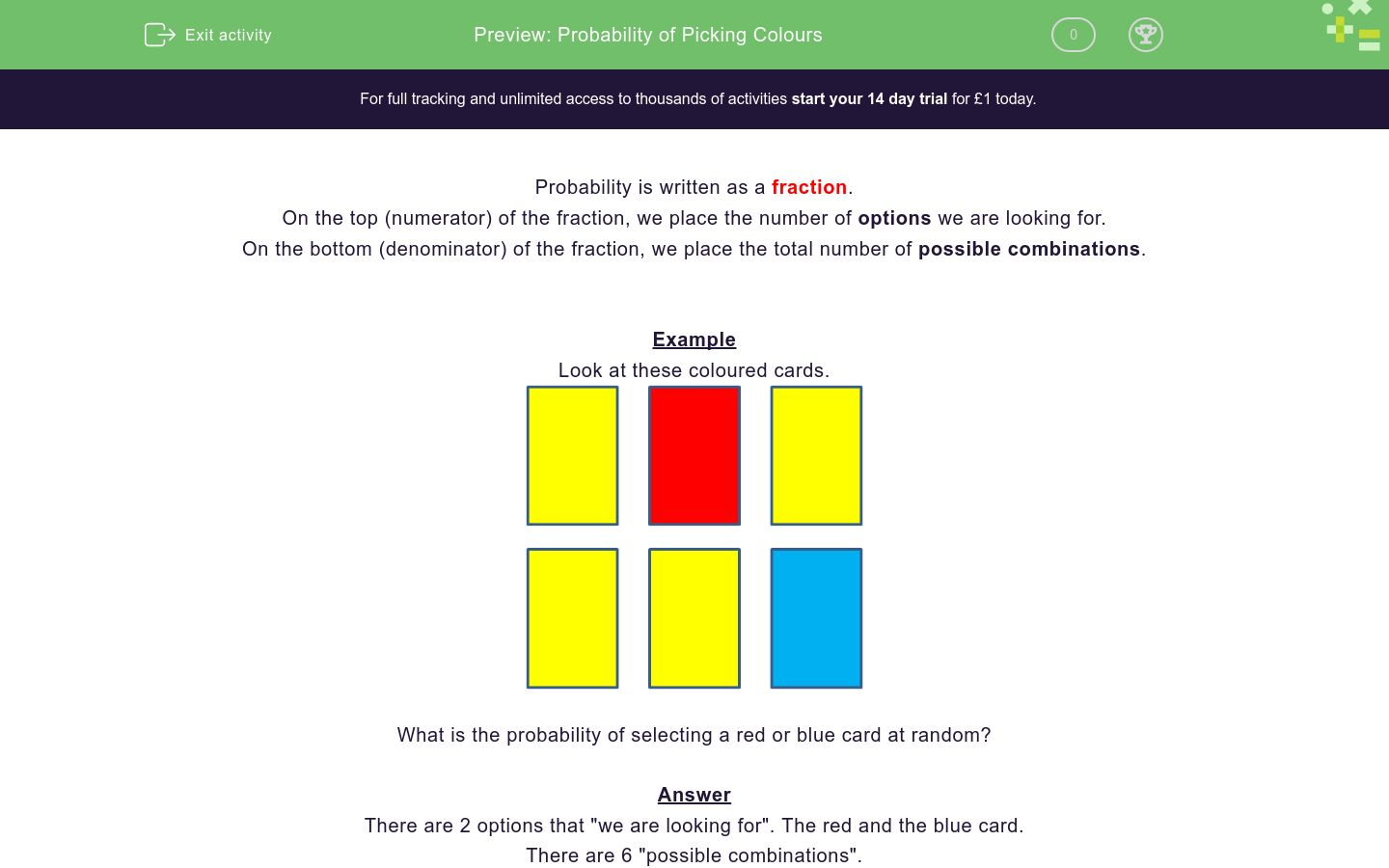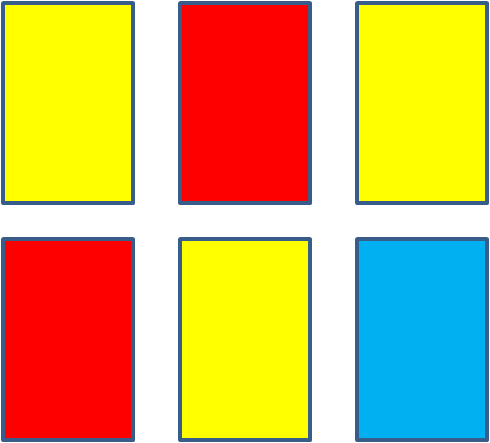# Probability of Picking Colours

In this worksheet, students calculate the probability of choosing or not choosing certain colours.Key stage:  KS 3

Curriculum topic:   Probability

Curriculum subtopic:   Record, Describe and Analyse Probability Experiments

Difficulty level:### QUESTION 1 of 10

Probability is written as a fraction.

On the top (numerator) of the fraction, we place the number of options we are looking for.

On the bottom (denominator) of the fraction, we place the total number of possible combinations.

Example

Look at these coloured cards.What is the probability of selecting a red or blue card at random?

There are 2 options that "we are looking for". The red and the blue card.

There are 6 "possible combinations".

The probability of choosing a yellow card is 2/6 which reduces to 1/3.

Look at these coloured cards.What is the probability of selecting a red or yellow card at random?

(write the answer as a fraction in its lowest terms using the / sign e.g. 3/4)

Look at these coloured cards.What is the probability of selecting a red or blue card at random?

(write the answer as a fraction in its lowest terms using the / sign e.g. 3/4)

Look at these coloured cards.What is the probability of selecting a blue or yellow card at random?

(write the answer as a fraction in its lowest terms using the / sign e.g. 3/4)

Look at these coloured cards.What is the probability of selecting a card at random which is not red?

(write the answer as a fraction in its lowest terms using the / sign e.g. 3/4)

Look at these coloured cards.What is the probability of selecting a green card at random?

(write the answer as a fraction in its lowest terms using the / sign e.g. 3/4)

Look at these coloured cards.What is the probability of selecting a red or blue card at random?

(write the answer as a fraction in its lowest terms using the / sign e.g. 3/4)

Look at these coloured cards.What is the probability of selecting a yellow or red card at random?

(write the answer as a fraction in its lowest terms using the / sign e.g. 3/4)

Look at these coloured cards.What is the probability of selecting a card at random which is not red?

(write the answer as a fraction in its lowest terms using the / sign e.g. 3/4)

Look at these coloured cards.What is the probability of selecting a blue or yellow card at random?

(write the answer as a fraction in its lowest terms using the / sign e.g. 3/4)

Look at these coloured cards.What is the probability of selecting a card at random which is not yellow?

(write the answer as a fraction in its lowest terms using the / sign e.g. 3/4)

• Question 1

Look at these coloured cards.What is the probability of selecting a red or yellow card at random?

(write the answer as a fraction in its lowest terms using the / sign e.g. 3/4)

5/6
• Question 2

Look at these coloured cards.What is the probability of selecting a red or blue card at random?

(write the answer as a fraction in its lowest terms using the / sign e.g. 3/4)

1/2
• Question 3

Look at these coloured cards.What is the probability of selecting a blue or yellow card at random?

(write the answer as a fraction in its lowest terms using the / sign e.g. 3/4)

2/3
• Question 4

Look at these coloured cards.What is the probability of selecting a card at random which is not red?

(write the answer as a fraction in its lowest terms using the / sign e.g. 3/4)

2/3
• Question 5

Look at these coloured cards.What is the probability of selecting a green card at random?

(write the answer as a fraction in its lowest terms using the / sign e.g. 3/4)

0
EDDIE SAYS
0/6 = 0
• Question 6

Look at these coloured cards.What is the probability of selecting a red or blue card at random?

(write the answer as a fraction in its lowest terms using the / sign e.g. 3/4)

5/8
• Question 7

Look at these coloured cards.What is the probability of selecting a yellow or red card at random?

(write the answer as a fraction in its lowest terms using the / sign e.g. 3/4)

3/4
• Question 8

Look at these coloured cards.What is the probability of selecting a card at random which is not red?

(write the answer as a fraction in its lowest terms using the / sign e.g. 3/4)

5/8
• Question 9

Look at these coloured cards.What is the probability of selecting a blue or yellow card at random?

(write the answer as a fraction in its lowest terms using the / sign e.g. 3/4)

2/3
• Question 10

Look at these coloured cards.What is the probability of selecting a card at random which is not yellow?

(write the answer as a fraction in its lowest terms using the / sign e.g. 3/4)

5/6
---- OR ----

Sign up for a £1 trial so you can track and measure your child's progress on this activity.

### What is EdPlace?

We're your National Curriculum aligned online education content provider helping each child succeed in English, maths and science from year 1 to GCSE. With an EdPlace account you’ll be able to track and measure progress, helping each child achieve their best. We build confidence and attainment by personalising each child’s learning at a level that suits them.

Get started